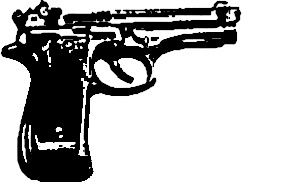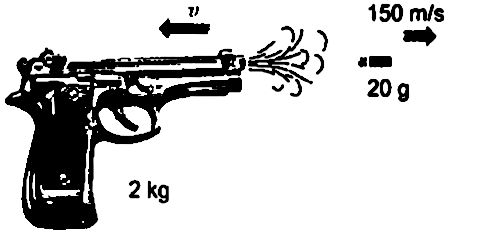Home/Class 9/Physics/

A bullet of mass $$20\, g$$ is horizontally fired with a horizontal velocity $$150\,ms^{-1}$$ from a pistol of mass $$2\, kg$$. What is the recoil velocity of the pistol?Speed
00:00
04:19## QuestionPhysicsClass 9

A bullet of mass $$20\, g$$ is horizontally fired with a horizontal velocity $$150\,ms^{-1}$$ from a pistol of mass $$2\, kg$$. What is the recoil velocity of the pistol?

As per question-
Mass of bullet, $$m_b=20\,g=0.02\,kg$$
Mass of pistol, $$m_p=2\,kg$$
Initial velocity of bullet, $$u_b=0$$
Final velocity of bullet, $$v_b=150\,ms^{-1}$$
Initial velocity of pistol, $$u_b=0$$
Now let us find out momentum before firingAs, momentum $$p=mv$$
So, $$p_1=m_bu_b+m_pu_p$$
$$=0.02\times 0+2\times 0$$
$$p_1=0\ kg\ m\ s^{-1}$$
Now, let us find out momentum after firing$$p=m_pv_p+m_bv_b$$
$$=2\times v_p+0.02\times 150$$
$$p_2=3+2v_p$$
From law of conservation of momentum,
Momentum after firing $$=$$Momentum before firing
$$3+2v_p=0$$
So, we get $$v_p=-1.5\,ms^{-1}$$
Here, negative sign denotes that the direction in which the pistol would recoil is opposite to that of bullet.